##### Physics I For Dummies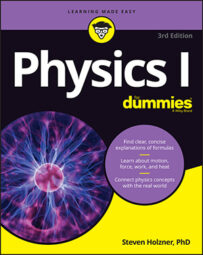In physics, you can connect the impulse you give to an object — like striking a pool ball with a cue — with the object’s change in momentum; all you need is a little algebra and a process called the impulse-momentum theorem.

What makes the connection easy is that you can play with the equations for impulse and momentum to simplify them so you can relate the two topics. What equations does physics have in its arsenal to connect these two? Relating force and velocity is a start. For example, force equals mass times acceleration, and the definition of average acceleration is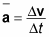where v stands for velocity and t stands for time. Now you may realize that if you multiply that equation by the mass, you get force, which brings you closer to working with impulse: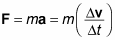Now you have force in the equation. To get impulse, multiply the force equation by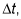the time over which you apply the force: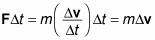Take a look at the final expression,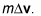Because momentum equals mv, this is just the difference in the object’s initial and final momentum: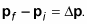Therefore, you can add that to the equation: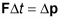Now take a look at the term on the left,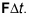That’s the impulse, J, or the average force applied to the object multiplied by the time that force was applied. Therefore, you can write this equation as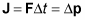Getting rid of everything in the middle finally gives you the impulse-momentum theorem, which says that impulse equals change in momentum: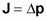The impulse-momentum theorem defines a very simple relation between the impulse and momentum, namely that impulse is equal to the change in momentum. You can also see how a constant or average force applied over a time is equal to an impulse that is given by the force multiplied by the time: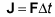Last, you can tie the force and momentum together through the impulse, which gives you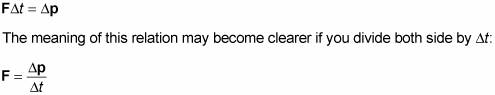So you see that the force is given by the rate of change of momentum. This is a whole new way of thinking about force! Wherever you see momentum changing with time, you know a force is acting, and if calculating the momentum is easier, it can lead to an easier way of calculating the force.